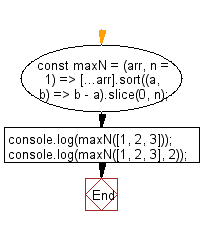# JavaScript: Get the n maximum elements from the provided array

## JavaScript fundamental (ES6 Syntax): Exercise-87 with Solution

Write a JavaScript program to get the n maximum elements from the provided array. If n is greater than or equal to the provided array's length, then return the original array(sorted in descending order).

• Use Array.prototype.sort() combined with the spread operator (...) to create a shallow clone of the array and sort it in descending order.
• Use Array.prototype.slice() to get the specified number of elements.
• Omit the second argument, n, to get a one-element array.
• If n is greater than or equal to the provided array's length, then return the original array (sorted in descending order).

Sample Solution:

JavaScript Code:

``````//#Source https://bit.ly/2neWfJ2
const maxN = (arr, n = 1) => [...arr].sort((a, b) => b - a).slice(0, n);
console.log(maxN([1, 2, 3]));
console.log(maxN([1, 2, 3], 2));
```
```

Sample Output:

```
[3,2]
```

Pictorial Presentation:Flowchart:Live Demo:

See the Pen javascript-basic-exercise-87-1 by w3resource (@w3resource) on CodePen.

Improve this sample solution and post your code through Disqus

What is the difficulty level of this exercise?

Test your Programming skills with w3resource's quiz.

﻿

## JavaScript: Tips of the Day

Chunks an array into n smaller arrays

Example:

```const tips_chunkIntoN = (arr, n) => {
const size = Math.ceil(arr.length / n);
return Array.from({ length: n }, (v, i) =>
arr.slice(i * size, i * size + size)
);
}
console.log(tips_chunkIntoN([1, 2, 3, 4, 5, 6, 7,8], 4));
```

Output:

```[[1,2],[3,4],[5,6],[7,8]]
```